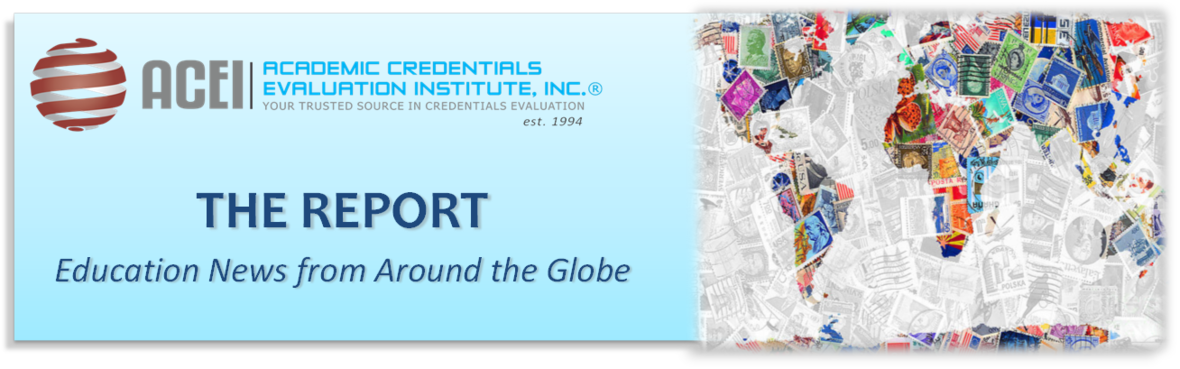# February 2020 - Beverly Hills, CA

ACADEMIC CREDENTIALS EVALUATION INSTITUTE, INC. (ACEI)
Founded in 1994, ACEI is a full-service company providing complete and integrated services in-house in the areas of international education research, credential evaluation, and translation. And, through our Global Consulting Group®, we offer our expertise in the following specialties: Media and Branding, Global Pathways, and Diversity, Equity and Inclusion (DEI) to interested institutions and organizations around the globe.

Subscribe to our BLOG

 table div table+table+table div table{width:100%;padding:0}table div table+table+table div table img{width:96.23%;padding:0;float:none}table div table+table+table div table td{width:100%;padding:0 1.88% 18px}/* styles */table div table+table+table+table+table+table div table{width:100%;padding:0}table div table+table+table+table+table+table div table img{width:96.23%;padding:0;float:none}table div table+table+table+table+table+table div table td{width:100%;padding:0 1.88% 18px}/* styles */# News Around the world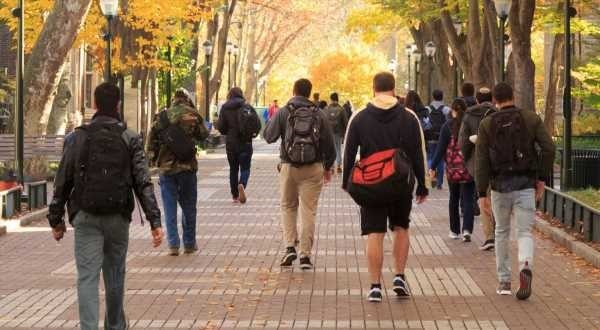# SAT for Chinese Students Scrapped, for now

Chinese students’ plans to study in foreign schools and universities are being dashed with key entrance exams being scrapped amid the ongoing coronavirus outbreak. To read more, click here.

 table div table+table+table+table+table+table+table+table+table+table div table{width:100%;padding:0}table div table+table+table+table+table+table+table+table+table+table div table img{width:96.23%;padding:0;float:none}table div table+table+table+table+table+table+table+table+table+table div table td{width:100%;padding:0 1.88% 18px}/* styles */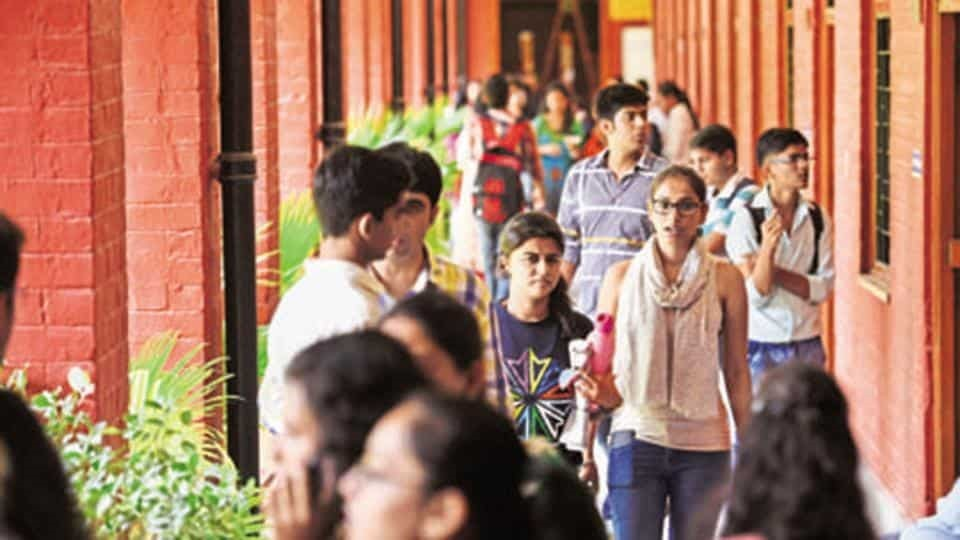Image credit: Saumya Khandelwal/HT

# Landscape of India’s Higher Education in the Near Future

Over the next five years, it is estimated that three out of 10 Indians will be in the age group of 18-22, the ages that need education and employment. With 119 million young Indians, the current education system will be inadequate. To read more, click here.

 table div table+table+table+table+table+table+table+table+table+table+table+table+table div table{width:100%;padding:0}table div table+table+table+table+table+table+table+table+table+table+table+table+table div table img{width:96.23%;padding:0;float:none}table div table+table+table+table+table+table+table+table+table+table+table+table+table div table td{width:100%;padding:0 1.88% 18px}/* styles */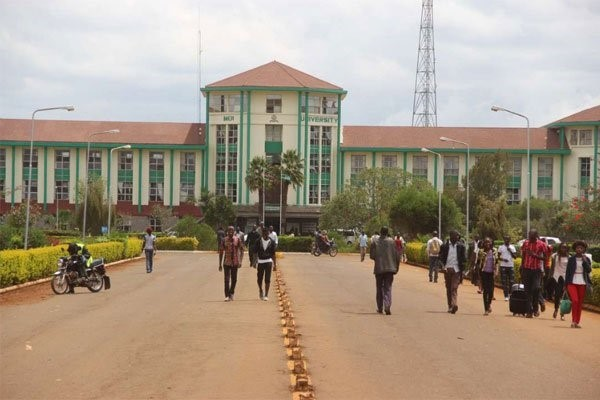Image credit: NATION MEDIA GROUP

# Moi University Closes 2 Campuses

A special committee at Moi University has recommended the closure of two satellite campuses due to lack of students. To read more, click here.

 table div table+table+table+table+table+table+table+table+table+table+table+table+table+table+table+table div table{width:100%;padding:0}table div table+table+table+table+table+table+table+table+table+table+table+table+table+table+table+table div table img{width:96.23%;padding:0;float:none}table div table+table+table+table+table+table+table+table+table+table+table+table+table+table+table+table div table td{width:100%;padding:0 1.88% 18px}/* styles */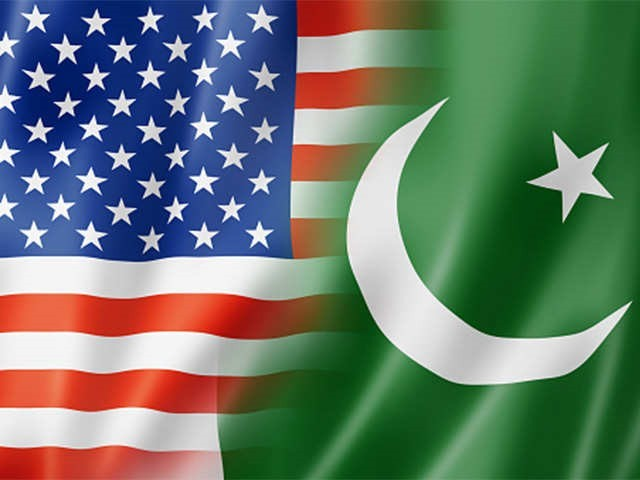# Rising Pakistani Interest in U.S. Higher Education

Amid rising Pakistani interest in U.S. higher education, representatives from 13 U.S. universities met thousands of Pakistani students during visits to schools, universities, and college fairs in Islamabad, Lahore, and Karachi as part of EducationUSA’s 16th South Asia Tour. To read more, click here.

 table div table+table+table+table+table+table+table+table+table+table+table+table+table+table+table+table+table+table div table{width:100%;padding:0}table div table+table+table+table+table+table+table+table+table+table+table+table+table+table+table+table+table+table div table img{width:96.23%;padding:0;float:none}table div table+table+table+table+table+table+table+table+table+table+table+table+table+table+table+table+table+table div table td{width:100%;padding:0 1.88% 18px}/* styles */# Putin Demands Closure of “Dud” Universities

Russian President Vladimir Putin has instructed the national government to move as quickly as possible to close inefficient domestic universities, particularly those which provide low-quality education to their students. To read more, click here.

 table div table+table+table+table+table+table+table+table+table+table+table+table+table+table+table+table+table+table+table+table div table{width:100%;padding:0}table div table+table+table+table+table+table+table+table+table+table+table+table+table+table+table+table+table+table+table+table div table img{width:96.23%;padding:0;float:none}table div table+table+table+table+table+table+table+table+table+table+table+table+table+table+table+table+table+table+table+table div table td{width:100%;padding:0 1.88% 18px}/* styles */Image credit: Delwyn Verasamy

# The Poor Receive Poorer Schooling

Attention has been given to democratic South Africa’s first matric pass rate of more than 80%, but the reality is that schools with a 0% pass rate still exist. To read more, click here.

 table div table+table+table+table+table+table+table+table+table+table+table+table+table+table+table+table+table+table+table+table+table+table+table div table{width:100%;padding:0}table div table+table+table+table+table+table+table+table+table+table+table+table+table+table+table+table+table+table+table+table+table+table+table div table img{width:96.23%;padding:0;float:none}table div table+table+table+table+table+table+table+table+table+table+table+table+table+table+table+table+table+table+table+table+table+table+table div table td{width:100%;padding:0 1.88% 18px}/* styles */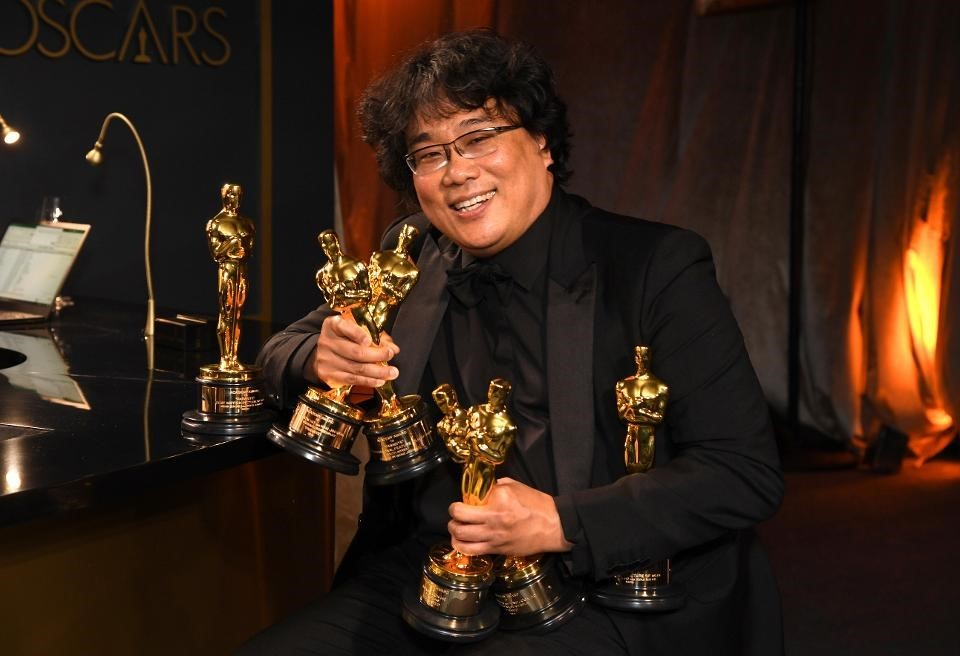Image credit: Richard Shotwell/Invision/AP

# What “Parasite” Tells Us about Higher Education and Inequality

This year’s Academy Award for Best Picture went to Parasite, a South Korean film that touched on themes of inequality and class conflict. One character forges a credential “proving” his enrollment in a university in order to land a job as a tutor. The plot point underscores the idea that in South Korea and the rest of the developed world, a college education is the only way to get ahead. To read more, click here.

 table div table+table+table+table+table+table+table+table+table+table+table+table+table+table+table+table+table+table+table+table+table+table+table+table+table+table div table{width:100%;padding:0}table div table+table+table+table+table+table+table+table+table+table+table+table+table+table+table+table+table+table+table+table+table+table+table+table+table+table div table img{width:96.23%;padding:0;float:none}table div table+table+table+table+table+table+table+table+table+table+table+table+table+table+table+table+table+table+table+table+table+table+table+table+table+table div table td{width:100%;padding:0 1.88% 18px}/* styles */# Making Music Education Accessible to Rich and Poor

 table div table+table+table+table+table+table+table+table+table+table+table+table+table+table+table+table+table+table+table+table+table+table+table+table+table+table+table+table+table div table{width:100%;padding:0}table div table+table+table+table+table+table+table+table+table+table+table+table+table+table+table+table+table+table+table+table+table+table+table+table+table+table+table+table+table div table img{width:96.23%;padding:0;float:none}table div table+table+table+table+table+table+table+table+table+table+table+table+table+table+table+table+table+table+table+table+table+table+table+table+table+table+table+table+table div table td{width:100%;padding:0 1.88% 18px}/* styles */Image: courtesy KPMB Architects

# Boston University: Building the City’s Largest Carbon-Neutral, Fossil-Fuel-Free Building

Boston University’s new Center for Computing and Data Sciences, designed by KPMB Architects, will include a bevy of environmentally friendly initiatives. To read more, click here.

 table div table+table+table+table+table+table+table+table+table+table+table+table+table+table+table+table+table+table+table+table+table+table+table+table+table+table+table+table+table+table+table+table div table{width:100%;padding:0}table div table+table+table+table+table+table+table+table+table+table+table+table+table+table+table+table+table+table+table+table+table+table+table+table+table+table+table+table+table+table+table+table div table img{width:96.23%;padding:0;float:none}table div table+table+table+table+table+table+table+table+table+table+table+table+table+table+table+table+table+table+table+table+table+table+table+table+table+table+table+table+table+table+table+table div table td{width:100%;padding:0 1.88% 18px}/* styles */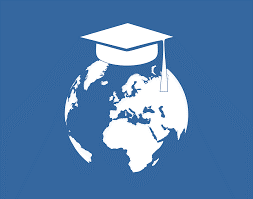# Rethinking Higher Education to Serve Equality and Sustainability

The United Nations Educational, Scientific and Cultural Organization-backed ‘Futures of Education’ initiative has called for the democratization of knowledge and a clear commitment by higher education and knowledge producing institutions to an agenda that primarily values equality and sustainability.

 table div table+table+table+table+table+table+table+table+table+table+table+table+table+table+table+table+table+table+table+table+table+table+table+table+table+table+table+table+table+table+table+table+table+table div table{width:100%;padding:0}table div table+table+table+table+table+table+table+table+table+table+table+table+table+table+table+table+table+table+table+table+table+table+table+table+table+table+table+table+table+table+table+table+table+table div table img{width:96.23%;padding:0;float:none}table div table+table+table+table+table+table+table+table+table+table+table+table+table+table+table+table+table+table+table+table+table+table+table+table+table+table+table+table+table+table+table+table+table+table div table td{width:100%;padding:0 1.88% 18px}/* styles */# Upcoming Events## Upcoming Events 2020:

SXSW EDU
March 9-12, 2020, Austin, TX
AICE 2020 Symposium
“Setting the Standard: Evaluating Professional Qualifications in Law and Medicine from Around the World.”
April 4, 2019, New Orleans, LA
AACRAO 2020 Annual Meeting
April 4-8, 2020, New Orleans, LA
Groningen Declaration Network Annual Meeting
April 15-17, 2020 Delhi, India
NAFSA Annual Conference
May 24-29, 2020, St. Louis, MO
EAIE Annual Conference
September 15-18, 2020, Barcelona, Spain
TAICEP Annual Conference
October 5-8, 2020, Glasgow, Scotland

 table div table+table+table+table+table+table+table+table+table+table+table+table+table+table+table+table+table+table+table+table+table+table+table+table+table+table+table+table+table+table+table+table+table+table+table+table+table+table div table{width:100%;padding:0}table div table+table+table+table+table+table+table+table+table+table+table+table+table+table+table+table+table+table+table+table+table+table+table+table+table+table+table+table+table+table+table+table+table+table+table+table+table+table div table img{width:96.23%;padding:0;float:none}table div table+table+table+table+table+table+table+table+table+table+table+table+table+table+table+table+table+table+table+table+table+table+table+table+table+table+table+table+table+table+table+table+table+table+table+table+table+table div table td{width:100%;padding:0 1.88% 18px}/* styles */table.module-39{width:84.72%;padding:0}table div table+table+table+table+table+table+table+table+table+table+table+table+table+table+table+table+table+table+table+table+table+table+table+table+table+table+table+table+table+table+table+table+table+table+table+table+table+table+table+table div table{width:84.72%;float:none;margin-left:auto;margin-right:auto;padding:0}table div table+table+table+table+table+table+table+table+table+table+table+table+table+table+table+table+table+table+table+table+table+table+table+table+table+table+table+table+table+table+table+table+table+table+table+table+table+table+table+table div table a{border:0 none;text-decoration:none}table div table+table+table+table+table+table+table+table+table+table+table+table+table+table+table+table+table+table+table+table+table+table+table+table+table+table+table+table+table+table+table+table+table+table+table+table+table+table+table+table div table img{width:100%!important;border:0 none;text-decoration:none}table div table+table+table+table+table+table+table+table+table+table+table+table+table+table+table+table+table+table+table+table+table+table+table+table+table+table+table+table+table+table+table+table+table+table+table+table+table+table+table+table div table td{width:100%;padding:0}/* styles */

# Digital Delivery of Evaluation Reports: SecurePathway©:

ACEI is a proud signatory of the Groningen Declaration Network which “seeks common ground in best serving the academic and professional mobility needs of citizens worldwide by bringing together key stakeholders in the Digital Student Data Ecosystem.” For the past two years, ACEI has committed itself to this shared mission through the introduction of SecurePathway©, an ACEI proprietary digital platform for the secure delivery of official evaluation reports and supporting educational credentials. For information on ACEI’s SecurePathway©.

Signing up with ACEI’s SecurePathway©, is free and does not cost the institutions any fees. To receive ACEI expert reports and certified academic credentials via SecurePathway©, complete this form.

*SecurePathway Registration Form*/* styles */ ACEI’s Advisory Services provide institutions and organizations looking at expanding their international student recruitment with customized solutions to help overcome enrollment challenges, uncover opportunities, and devise an innovative and holistic approach to international enrollment. For more information on ACEI’s Advisory Services and how we can assist you, please contact Yolinisse Moreno, Director of Programs & Communications at ymoreno@acei-global.org.
 table div table+table+table+table+table+table+table+table+table+table+table+table+table+table+table+table+table+table+table+table+table+table+table+table+table+table+table+table+table+table+table+table+table+table+table+table+table+table+table+table+table+table+table+table div table{width:100%;padding:0}table div table+table+table+table+table+table+table+table+table+table+table+table+table+table+table+table+table+table+table+table+table+table+table+table+table+table+table+table+table+table+table+table+table+table+table+table+table+table+table+table+table+table+table+table div table img{width:96.23%;padding:0;float:none}table div table+table+table+table+table+table+table+table+table+table+table+table+table+table+table+table+table+table+table+table+table+table+table+table+table+table+table+table+table+table+table+table+table+table+table+table+table+table+table+table+table+table+table+table div table td{width:100%;padding:0 1.88% 18px}/* styles *//* styles */ ACEI offers a comprehensive range of professional services that include: international credential evaluations; translations; consulting; and training. For more information on ACEI, please visit www.acei-global.org or contact: Tel: 1-310-275-3530; Email: acei@acei-global.org. ACEI was founded and incorporated in 1994 in the State of California and is a Charter and Endorsed Member of the Association of International Credential Evaluators (AICE).
 Like   Tweet   Pin   +1   in